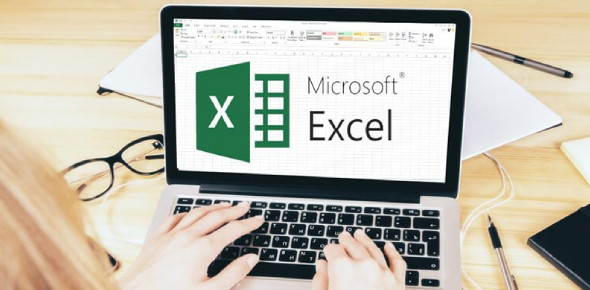# Test Your Knowledge: Microsoft Excel Quiz!

20 Questions | Total Attempts: 3224SettingsWhat do you know about Microsoft Excel, and do you think you can pass this quiz? Some people are skilled when it comes to computers, while others struggle to figure them out. Luckily, Microsoft Excel has useful features that anyone can appreciate. It reads like a spreadsheet, in which you can organize items into columns and rows. IF you want to learn more about Microsoft Excel, look no further than this quiz.

• 1.
Excel is an electronic version of a(n)
• A.

Database

• B.

• C.

Expression

• D.

Formula

• 2.
Excel stores its documents as files called
• A.

Worksheets

• B.

Sheet tabs

• C.

Workbooks

• D.

Books

• 3.
A(n) ____ is a group of cells.
• A.

Cluster

• B.

• C.

Selection

• D.

Range

• 4.
The characters +, -, *, and / are examples of:
• A.

Formulas

• B.

Values

• C.

Arithmetic operators

• D.

Calculations

• 5.
The formula used to multiply cell A1 by cell C1 is ____.
• A.

=A1*C1

• B.

C1*A1

• C.

=A1/C1

• D.

A1*C1

• 6.
Formatting changes only the appearance of data - it does not affect the data itself.
• A.

True

• B.

False

• 7.
You can use a picture or image as the background for all the cells in a worksheet.
• A.

True

• B.

False

• 8.
Background images do not print in Excel.
• A.

True

• B.

False

• 9.
When using a data bar, changing the value of one cell in the range will not affect the size of all the other cells’ data bars.
• A.

True

• B.

False

• 10.
In Excel, if the built-in styles don’t meet your needs, you can modify an existing style or create a new one.
• A.

True

• B.

False

• 11.
The PMT function is a(n) ____ function.
• A.

Date

• B.

IF

• C.

Financial

• D.

Statistical

• 12.
The F FV function stands for ____.
• A.

Function value

• B.

Future value

• C.

Financial value

• D.

Formulated value

• 13.
Absolute references are marked with a ____.
• A.

• B.

:

• C.

\$

• D.

!

• 14.
When you copy a formula that contains an absolute reference to a new location, the reference ____.
• A.

Is updated automatically

• B.

Does not change

• C.

Becomes bold

• D.

Has a dotted outline in its cell

• 15.
Which function counts how many cells in a range contain numbers, where value1, value2, and so forth are text, numbers, or cell references?
• A.

SUM

• B.

COUNT

• C.

COUNTA

• D.

AUTOSUM

• 16.
Every chart must have a data source.
• A.

True

• B.

False

• 17.
For  which of the following reasons would you choose to use a chart or graph?
• A.

To organize information in columns and rows

• B.

To show trends or relationships across data

• C.

To present data using text only

• D.

To add color to a report

• 18.
To move or resize an embedded chart, ____.
• A.

Select the chart and drag the selection box

• B.

Drag the resize handle on the selection box

• C.

Select the chart and press Tab

• D.

Both a and b

• 19.
A(n) ____________________ is a visual representation of a set of data.
• A.

Chart

• B.

Graph

• 20.
Excel supports ____________________ built-in charts.
• A.

73

• B.

75

Related TopicsBack to top